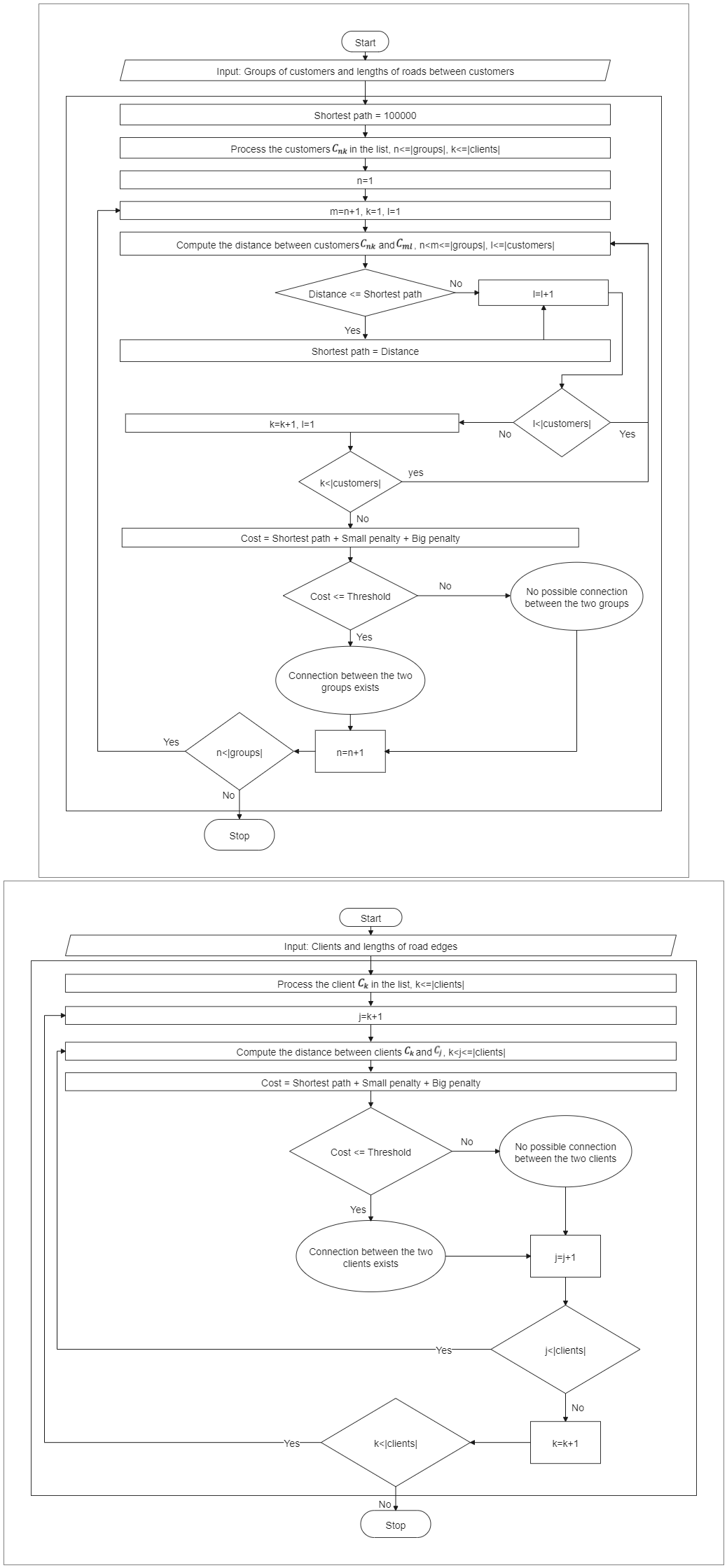Template Community / Shortest Path Flowchart

# Shortest Path Flowchart

Share
Duplicate0
0
Report
Publish time：11-28-2021
The shortest part flowchart is the problem of finding a path between two vertices in a graph such that the sum of the weights of its constituent edges is minimized. As shown here, Dijkstra's algorithm can determine the shortest path from one node in a graph to every other node within the same graph data structure, provided that the nodes are reachable from the starting node. In simpler words, the problem of finding the shortest path between two intersections on a road map may be modeled as a special case of the shortest path problem in graphs, where the vertices correspond to intersections, and the edges correspond to road segments, each weighted by the length of the segment.
Creator